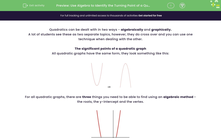# Use Algebra to Identify the Turning Point of a Quadratic

In this worksheet, students will practise completing the square to find the turning point (vertex) of a quadratic graph.Key stage:  KS 4

Year:  GCSE

GCSE Subjects:   Maths

GCSE Boards:   AQA, Eduqas, OCR, Pearson Edexcel,

Curriculum topic:   Algebra, Graphs of Equations and Functions

Curriculum subtopic:   Graphs Graphs of Equations and Functions

Popular topics:   Square Numbers worksheets

Difficulty level:#### Worksheet Overview

Quadratics can be dealt with in two ways - algebraically and graphically.

A lot of students see these as two separate topics, however, they do cross over and you can use one technique when dealing with the other.

The significant points of a quadratic graph

All quadratic graphs have the same form, they look something like this:For all quadratic graphs, there are three things you need to be able to find using an algebraic method - the roots, the y-intercept and the vertex.In this worksheet, we’re going to look at how we find the vertex (the turning point) algebraically.

Example:

How to find the coordinates of the vertex (turning point) for the quadratic y = x2 + 3x - 4

For this, we have to use one of the techniques for dealing with quadratics, completing the square.

Step 1: Complete the square for the quadratic

Using the technique of completing the square, we can see that:

y = x2 + 3x – 4 → y = (x + 1.5)2 – 6.25

Step 2: Find the x  coordinate of the turning point

This relies on you knowing something about square numbers – they can never be negative.

The smallest a squared number can be is 0.

When we are looking for the vertex, we are looking for where the value of y is as low as possible (the minimum) - this happens when the squared bracket is zero.

So what value of x will make the squared bracket zero?

(x + 1.5)2 =  0

x + 1.5 =  0

x = -1.5

Step 3: Find the y  coordinate of the turning point

We’ve already worked out that the minimum point is at x = -1.5.

To find the value of y, all we have to do is to plug x = -1.5 into the completed square  y = (x + 1.5)2 – 6.25

If we do this, we get y = (-1.5 + 1.5)2 – 6.25

The bracket now becomes zero and leaves us with y = -6.25

This means that the coordinates of the vertex are (-1.5,-6.25)

Is the vertex always the lowest point?

No. If you have a graph of the form y = x2 + ax + b you will get a minimum, however if you have a graph of the form y = -x2 + ax + b, you will get a maximum.

Let's try some questions now.

### What is EdPlace?

We're your National Curriculum aligned online education content provider helping each child succeed in English, maths and science from year 1 to GCSE. With an EdPlace account you’ll be able to track and measure progress, helping each child achieve their best. We build confidence and attainment by personalising each child’s learning at a level that suits them.

Get started